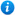* Watermarks do not appear on purchased resources

Preview resource: Click slides to enlarge

# Equivalent Fractions - Year 4

KS2 Maths Teaching Resources: Equivalent Fractions - Year 4

Year 4 maths programme of study - Number - fractions (including decimals):

• recognise and show, using diagrams, families of common equivalent fractions

In 'Equivalent Fractions - Year 4' pupils are taught how to recognise and show, using diagrams, equivalent fractions as per the year 4 curriculum objective listed above.

This maths teaching resource is an animated PowerPoint presentation with 7 accompanying worksheets designed to use with all abilities. Content includes:

• An introduction to fractions recap
• An explanation of equivalent fractions
• Name the equivalent fraction activity and worksheet
• Shading diagrams to represent the fraction and naming the equivalent fraction activity and worksheet
• An explanation of how to find equivalent fractions
• Finding equivalent fractions shading activity and worksheet
• Finding equivalent fractions activity and two accompanying worksheets
• The 1/2 Family, the 1/4 Family and the 1/10 Family of equivalent fractions
• Identifying the equivalent fractions of 1/2 activity and worksheet
• Identifying halves, thirds, quarters and sixths activity and worksheet
• Equivalent fractions cloud activity
• Equivalent fractions word problems and worksheet
• Link to an online equivalent fractions game
• Coloured fraction wall worksheets and black and white colouring sheets
• Cut out fraction wall strips
• Further differentiated equivalent fractions worksheet with answers

'Equivalent Fractions - Year 4' can be fully edited allowing teachers to adapt the resource to suit the individual needs of each class they teach.

To take a closer look at 'Equivalent Fractions - Year 4' please click on the PowerPoint images.

Our Price : £3.50 / 4 Credits